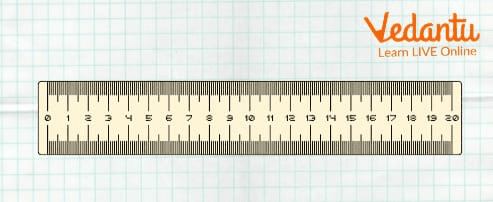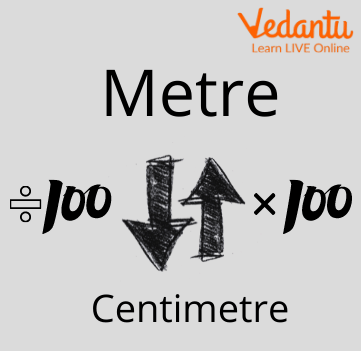Courses
Courses for Kids
Free study material
Free LIVE classes
More

# Metres Into CentimetresLIVE
Join Vedantu’s FREE Mastercalss

## Introduction

Just imagine that you are participating in a race competition with a group of friends and the winner is who runs fast. In order to decide who the winner is, you will have to measure the length. So you can measure this by using different types of units. One of the units is metre. We can calculate the length of the playground where the race competition happens with the help of a metre unit.Introduction of Metre and Centimetre

Metre: Metre is a primary unit of distance in the measuring system. In this, you will understand how to convert metre into centimetre.

Centimetre: A centimetre is a measuring unit of length that equals one-hundredth of a metre.

1m = 100cm.

Metre and centimetre are the units of distance that are measured with the help of scale or inch tape or any other thing where metre and centimetre are mentioned.

one metre is equal to 100 centimetres and 100 centimetres are equal to 1 metre.

We can say that the relation between metres and centimetres is reversed.

## Convert Metre to Centimetre

1 m = 100 cm

1 cm = 0.01 mScale

## Convert a Metre Into a Centimetre

We can convert metre to centimetre with the help of two steps that are given below:

Step 1: Multiply the number of metres by 100.

Step 2: change the units to centimetres.

For Example:

Convert 8 metres into centimetres.

8 m × 100 = 800

8 m = 800 cm.

Convert 1 metre to centimetres.

1 m × 100 = 100

1 m = 100 cm.

Just like we convert metres into centimetres, we can convert centimetres into metres. And the formula is just the opposite of this.

## Conversion of Centimetre Into Metre

We can convert centimetres into metres by following two steps that are :

Step 1: divide the centimetre number by 100

Step 2: change the unit to metres.

Remember the above example, the reverse of the first example where we convert 8 m to 800 cm. and we convert 800 cm to 8 m. Let's check through the example.

Example: Convert 800 cm to metres.

800 cm ÷ 100 = 8

800 cm = 8 m.Conversion

## Conversion Table

 Metre(m) Centimetre(cm) 0.01 m 1 cm 0.1 m 10 cm 1 m 100 cm 2 m 200 cm 3 m 300 cm 5 m 500 cm 10 m 1000 cm 20 m 2000 cm 50 m 5000 cm 100 m 10000 cm 1000 m 100000 cm

## Solved Example:

Q1. (a) How many centimetres are in a metre?

(b) One metre is equal to how many centimetres?

(c) Convert 0.7 m to cm

Sol: (a) 100 cm are in a metre.

(b) 1 m = 100 cm

(c) As we know 1 m = 100 cm

0.7 m = 0.7 x 100 cm = 70.0 cm = 70 cm

## Practice Questions

1. Convert 0.5 m to cm.

2. Convert 1000 cm to m.

3. Convert 550 cm to m.

4. Convert 75 m to cm.

5. Convert 885 cm to m.

Ans:

1. 50 cm

2. 10 m

3. 5.5 m

4. 7500 cm

5. 8.85 m.

## Summary

Metres and centimetres help to measure the length of any particular thing. The length is measured with the help of a scale. metre and centimetres are denoted on the scale. metre and centimetre are related to each other in a reversible manner.

1 m = 100 cm and 100 cm = 1 m.

Last updated date: 21st Sep 2023
Total views: 119.1k
Views today: 3.19k

## FAQs on Metres Into Centimetres

1. What do you mean by metre and centimetres and how can we convert the metre into a centimetre? Explain with an example.

A metre is a primary unit of length in the measuring system and Centimetre is a measuring unit of length that equals one-hundredth of a metre.

For conversion, we have to find the relationship between two units which is 1 m = 100 cm. By following the given steps, we can convert the metre to a centimetre.

Firstly, multiply the number by 100. After that change the unit to the centimetre.

Example:

Change the 65 metres into a centimetre.

1 m = 100 cm,

65 m = 65 × 100 = 6500 cm.

So the answer is 6500 cm.

2. What are the measuring tools of length and explain the relationship between metre and centimetre?

Length can be measured through various tools like measuring rod, scale, ruler, measuring tape, etc. A metre is a primary unit of length in the measuring system. We can convert a metre into a centimetre. A centimetre is a measuring unit of length that equals one-hundredth of a metre. One metre equals 100 centimetres and 100 centimetres is equal to 1 metre. There is a reverse relationship existing between metre and centimetre. Both are the units of measuring any length.